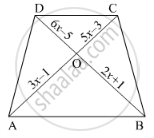Share

# In the Below Figure, If Ab || Cd, Find the Value of X. - Mathematics

Course

#### Question

In the below figure, If AB || CD, find the value of x.#### Solution

rArr(3x-1)/(5x-3)=(2x+1)/(6x-5)

⇒ (3x – 1) (6x – 5) = (2x + 1) (5x – 3)

⇒ 3x (6x – 5) – 1(6x – 5) = 2x (5x – 3) + 1 (5x – 3)

⇒ 18𝑥2 − 15𝑥 − 6𝑥 + 5 = 10𝑥2 − 6𝑥 + 5𝑥 − 3

⇒ 8𝑥2 − 20𝑥 + 8 = 0

⇒ 4(2𝑥2 − 5𝑥 + 2) = 0

⇒ 2𝑥2 − 4𝑥 − 1𝑥 + 2 = 0

⇒ 2𝑥(𝑥 − 2) − 1(𝑥 − 2) = 0

⇒ (2𝑥 − 1)(𝑥 − 2) = 0

⇒ 2x – 1 = 0 or x – 2 = 0

⇒ 𝑥 = 1/2 or 𝑥 = 2

𝑥 = 1/2 is not possible, because, OC = 5x – 3

= 5(1/2) - 3

=(5-6)/2=-1/2

Is there an error in this question or solution?

#### APPEARS IN

RD Sharma Solution for Class 10 Maths (2018 (Latest))
Chapter 7: Triangles
Ex. 7.4 | Q: 1.2 | Page no. 37
RD Sharma Solution for Class 10 Maths (2018 (Latest))
Chapter 7: Triangles
Ex. 7.4 | Q: 1.2 | Page no. 37

#### Video TutorialsVIEW ALL 

Solution In the Below Figure, If Ab || Cd, Find the Value of X. Concept: Triangles Examples and Solutions.
S